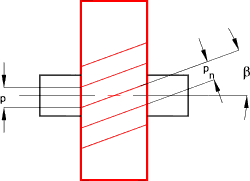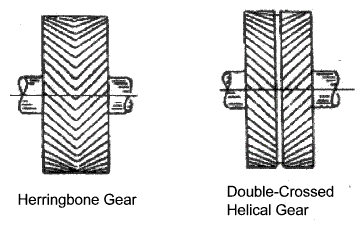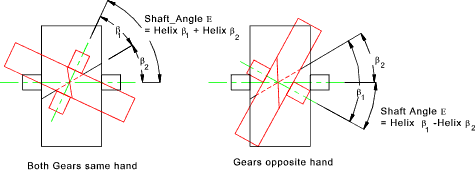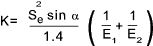Drive_Index
Gears Gearboxes

Helical Gears

Introduction

Helical gears are similar to spur gears except that the gears teeth are at an angle with the axis of the gears.  A helical gear is termed right handed or left handed as determined by the direction the teeth slope away from the viewer looking at the top gear surface along the axis of the gear.   ( Alternatively if a gear rests on its face the hand is in the direction of the slope of the teeth) .   Meshing helical gears must be of opposite hand. Meshed helical gears can be at an angle to each other (up to 90o ).  The helical gear provides a smoother mesh and can be operated at greater speeds than a straight spur gear.   In operatation helical gears generate axial shaft forces in addition to the radial shaft force generated by normal spur gears.

In operation the initial tooth contact of a helical gear is a point which develops into a full line contact as the gear rotates.   This is a smoother cycle than a spur which has an initial line contact.  Spur gears are generally not run at peripheral speed of more than 10m/s. Helical gears can be run at speed exceeding 50m/s when accurately machined and balanced.

Standards ... The same standards apply to helical gears as for spur gears
 AGMA 2001-C95 or AGMA-2101-C95 Fundamental Rating factors and Calculation Methods for involute Spur Gear and Helical Gear Teeth BS 436-4:1996, ISO 1328-1:1995..Spur and helical gears. Definitions and allowable values of deviations relevant to corresponding flanks of gear teeth BS 436-5:1997, ISO 1328-2:1997..Spur and helical gears. Definitions and allowable values of deviations relevant to radial composite deviations and runout information BS ISO 6336-1:1996 ..Calculation of load capacity of spur and helical gears. Basic principles, introduction and general influence factors BS ISO 6336-2:1996..Calculation of load capacity of spur and helical gears. Calculation of surface durability (pitting) BS ISO 6336-3:1996..Calculation of load capacity of spur and helical gears. Calculation of tooth bending strength BS ISO 6336-5:2003..Calculation of load capacity of spur and helical gears. Strength and quality of materials

Helical gear parameters

A helical gear train with parallel axes is very similar to a spur gear with the same tooth profile and proportions.  The primary difference is that the teeth are machined at an angle to the gear axis.Helix Angle ..

The helix angle of helical gears β is generally selected from the range 6,8,10,12,15,20 degrees. The larger the angle the smoother the motion and the higher speed possible however the thrust loadings on the supporting bearings also increases.   In case of a double or herringbone gear β values 25,30,35,40 degrees can also be used.  These large angles can be used because the side thrusts on the two sets of teeth cancel each other allowing larger angles with no penalty

Pitch /module ..

For helical gears the circular pitch is measured in two ways
The traverse circular pitch (p) is the same as for spur gears and is measured along the pitch circle
The normal circular pitch p n is measured normal to the helix of the gear.
The diametric pitch is the same as for spur gears     ... P = z g /dg = z p /d p ....d= pitch circle dia (inches).
The module is the same as for spur gears     ... m = dg/z g = d p/z p.... d = pitch circle dia (mm).

Helical Gear geometrical proportions
 p = Circular pitch = d g. p / z g = d p. p / z p p n = Normal circular pitch = p .cosβ P n =Normal diametrical pitch = P /cosβ p x = Axial pitch = p c /tanβ m n =Normal module = m / cosβ α n = Normal pressure angle = tan -1 ( tanα.cos β ) β =Helix angle d g = Pitch diameter gear = z g. m d p = Pitch diameter pinion = z p. m a =Center distance = ( z p + z g )* m n /2 cos β a a = Addendum = m a f =Dedendum = 1.25*m b = Face width of narrowest gear

Herringbone / double crossed helical gearsCrossed Helical Gears

When two helical gears are used to transmit power between non parallel, non-intersecting shafts, they are generally called crossed helical gears.  These are simply normal helical gears with non-parallel shafts.  For crossed helical gears to operate successfully they must have the same pressure angle and the same normal pitch.   They need not have the same helix angle and they do not need to be opposite hand.   The contact is not a good line contact as for parallel helical gears and is often little more than a point contact.  Running in crossed helical gears tend to marginally improve the area of contact.

The relationship between the shaft angles E and the helix angles β 1 & β2 is as follows

E = (Same Helix Angle) β 1 + β 2 ......(Opposite Helix Angle) β 1 - β 2For gears with a 90o crossed axis it is obvious that the gears must be the same hand.

The centres distance (a) between crossed helical gears is calculated as follows

a = m * [(z 1 / cos β 1) + ( z 1 / cos β 1 )] / 2

The sliding velocity Vsof crossed helical gears is given by

Vs = (V1 / cos β 1 ) = (V 2 / cos β 2 )

Strength and Durability calculations for Helical Gear Teeth

Designing helical gears is normally done in accordance with standards the two most popular series are listed under standards above: The notes below relate to approximate methods for estimating gear strengths. The methods are really only useful for first approximations and/or selection of stock gears (ref links below). � Detailed design of spur and helical gears should best be completed using :

a) Standards.
b) Books are available providing the necessary guidance.
c) Software is also available making the process very easy.    A very reasonably priced and easy to use package is included in the links below (Mitcalc.com)

The determination of the capacity of gears to transfer the required torque for the desired operating life is completed by determining the strength of the gear teeth in bending and also the durability i.e of the teeth ( resistance to wearing/bearing/scuffing loads ) .. The equations below are based on methods used by Buckingham..

Bending

The Lewis formula for spur gears can be applied to helical gears with minor adjustments to provide an initial conservative estimate of gear strength in bending.    This equation should only be used for first estimates.

σ = Fb / ( ba. m. Y )

 Fb = Normal force on tooth = Tangential Force Ft / cos β σ = Tooth Bending stress (MPa) ba = Face width (mm) Y = Lewis Form Factor m = Module (mm)

When a gear wheel is rotating the gear teeth come into contact with some degree of impact.  To allow for this a velocity factor is introduced into the equation.   This is given by the Barth equation for milled profile gears.

K v = (6,1 + V ) / 6,1

V = the pitch line velocity = PCD.w/2

The Lewis formula is thus modified as follows

σ = K v.Fb / ba. m. Y

The Lewis form factor Y must be determined for the virtual number of teeth z' = z /cos3β The bending stress resulting should be less than the allowable bending stress Sb for the gear material under consideration.   Some sample values are provide on this page ef Gear Strength Values

Surface Strength

The allowable gear force from surface durability considerations is determined approximately using the simple equation as follows

Fw = K v d p b a Q K / cos2βQ = 2. dg /( dp + dp ) = 2.zg /( zp +zp )

Fw = The allowable gear load. (MPa)

K = Gear Wear Load Factor (MPa) obtained by look up ref Gear Strength Values

 Lewis Form factor for Teeth profile α = 20o , addendum = m, dedendum = 1.25m Number of teeth Y Number of teeth Y Number of teeth Y Number of teeth Y Number of teeth Y 12 0.245 17 0.303 22 0.331 34 0.371 75 0.435 13 0.261 18 0.309 24 0.337 38 0.384 100 0.447 14 0.277 19 0.314 26 0.346 45 0.401 150 0.460 15 0.290 20 0.322 28 0.353 50 0.409 300 0.472 16 0.296 21 0.328 30 0.359 60 0.422 Rack 0.485

 Links to Gear Design Gear Design ...A comprehensive source of Gear Design Information Efunda ...Efunda -> Design Centre-> Gears.. Some useful Notes. Gear Design Topics ... A site providing amazing motion graphics of different gear types SEW Eurodrive...All the information on Gearboxes you will need Quality Transmission Components...Supplier with downloadable Gear Design Handbook Stock Drive Products= Sterling Instruments...Supplier with large quantity of downloadable drive information Mitcalc...Excel based software including coded gear design Lenze...Drive system supplier with geared motor section Artec Toothing Design...A number of short papers including on gears including helical gear teeth QTC helical gears...A number of helical gear specifications

Drive_Index
Gears Gearboxes